Home | | Maths 12th Std | Exercise 3.1: Vieta’s Formulae and Formation of Polynomial Equations

# Exercise 3.1: Vieta’s Formulae and Formation of Polynomial Equations

Maths Book back answers and solution for Exercise questions - Mathematics : Theory of Equations: Vieta’s Formulae and Formation of Polynomial Equations : Exercise problems with Questions, Answers, Solution, Explanation

EXERCISE 3.1

1. If the sides of a cubic box are increased by 1, 2, 3 units respectively to form a cuboid, then the volume is increased by 52 cubic units. Find the volume of the cuboid.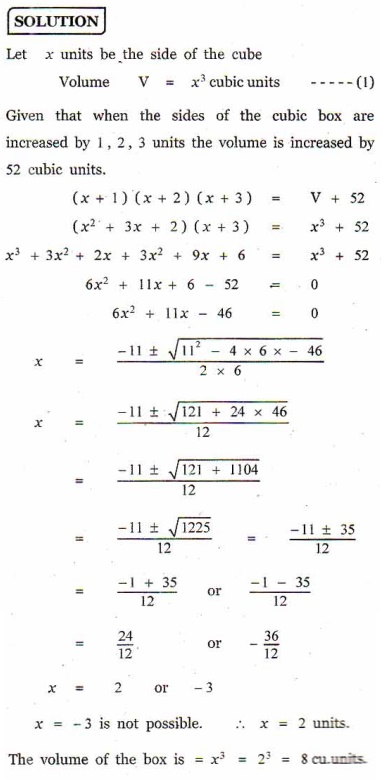2. Construct a cubic equation with roots

(i)  1, 2 , and 3 (ii)  1,1, and -2 (iii) 2, 1/2 and 13. If α β and γ are the roots of the cubic equation  x3 + 2x2 + 3+ 4 = 0 , form a cubic equation whose roots are

(i) 2α , 2β , 2γ

(ii) 1/α, 1/β, 1/γ

(iii) −α , −β , −γ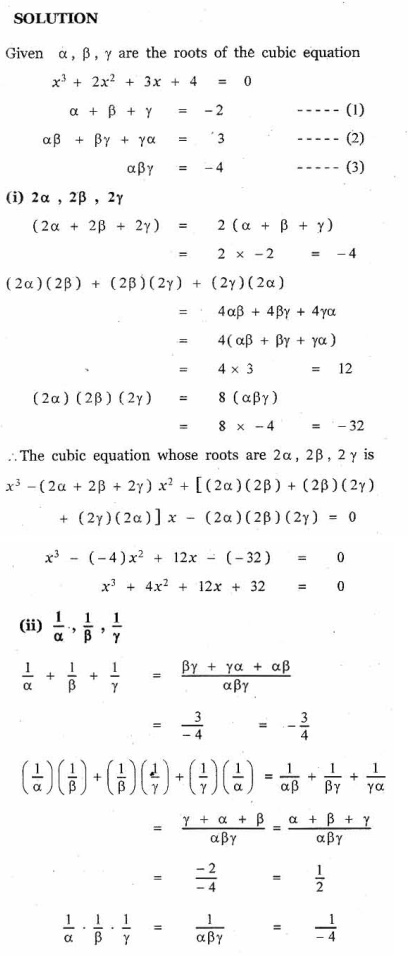4. Solve the equation 3x3 - 16x2 + 23- 6 = 0 if the product of two roots is 1.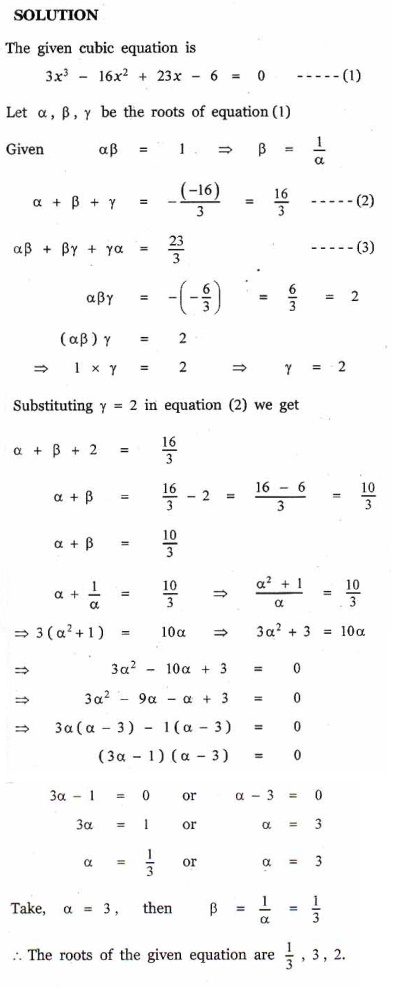5. Find the sum of squares of roots of the equation 2x4 - 8x3 + 6x2 - 3 = 0 .6. Solve the equation x3 - 9x2 +14+ 24 = 0 if it is given that two of its roots are in the ratio 3 : 2 .7. If α β , and γ are the roots of the polynomial equation ax3 + bx2 + cx = 0 , find the value ofin terms of the coefficients.8. If α β ,γ , and δ are the roots of the polynomial equation 2x4 + 5x3 - 7x2 + 8 = 0, find a quadratic equation with integer coefficients whose roots are α β γ δ and αβγδ .9. If and are the roots of the equation lx2 + nx = 0 , show that10. If the equations x2 + px = 0 and x2 + p’x q’ = 0 have a common root, show that it must be equal to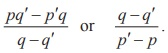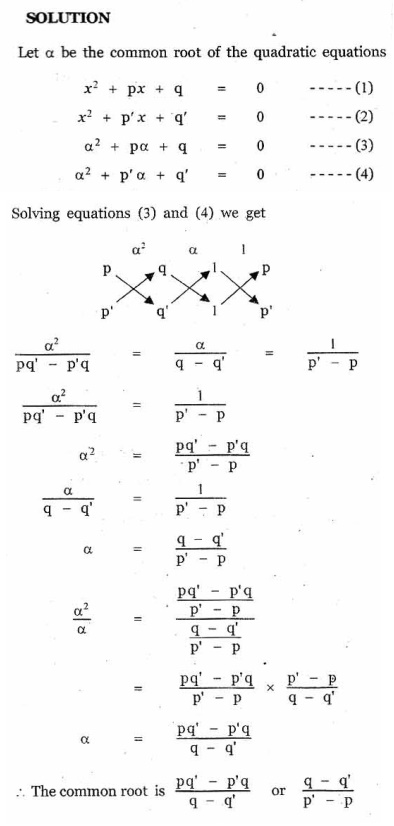11. Formalate into a mathematical problem to find a number such that when its cube root is added to it, the result is 6 .12. A 12 metre tall tree was broken into two parts. It was found that the height of the part which was left standing was the cube root of the length of the part that was cut away. Formulate this into a mathematical problem to find the height of the part which was cut away.